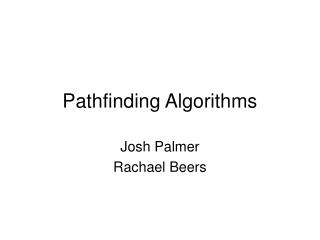Download PresentationPathfinding Algorithms

# Pathfinding Algorithms - PowerPoint PPT PresentationDownload Presentation## Pathfinding Algorithms

- - - - - - - - - - - - - - - - - - - - - - - - - - - E N D - - - - - - - - - - - - - - - - - - - - - - - - - - -
##### Presentation Transcript

1. Pathfinding Algorithms Josh Palmer Rachael Beers

2. Outline • Dijkstra’s Algorithm • Best-First Search • A*

3. Dijkstra’s • Visits vertices starting from starting point • Looks at all closest vertices to the starting point • From these points, we check the closest neighbors • Stop checking once goal is reached • Guaranteed shortest path • Time consuming

4. Dijkstra’s

5. Best-First Search • Similar to Dijkstra’s • Difference: Has a heuristic (estimate) to tell it how far the goal is from any vertex • Selects vertex closest to the goal instead of closest to starting point • Does not guarantee shortest path • Very fast

6. Best-first search

7. g(n) = distance from start to a vertex n • Dijkstra’s uses a g(n) measure • Almost an exhaustive approach • h(n) = estimated cost from a vertex n to the goal • Best-first search uses an h(n) measure • A heuristically informed approach

8. With an Obstacle Djikstra’s Best-first

9. A* • Combines the use of g(n) and h(n) • f(n) = g(n) + h(n) • A* finds the vertex n with the lowest f(n) • Continues to find vertices with the lowest f(n) until the goal is reached • Guarantees shortest path • Relatively fast

10. A*

11. A* with an obstacle

12. Other pathfinding algorithms • Breadth-first search and depth-first search • Many versions of A* • Lowest cost first • Heuristic depth-first • Iterative deepening • Bidirectional • Recursive best-first

13. References • Amit’s A*pages (images from here) http://theory.stanford.edu/~amitp/GameProgramming/ • AI Depot http://aidepot.com/Tutorial/PathFinding.html • Game AI Page http://www.gameai.com/pathfinding.html • A* algorithm tutorial (code from here) http://www.geocities.com/jheyesjones/astar.html • Games++: Games and Game Programming http://www.gamespp.com/algorithms/pathfinding • A* Pathfinding for Beginners http://www.policyalmanac.org/games/aStarTutorial.htm